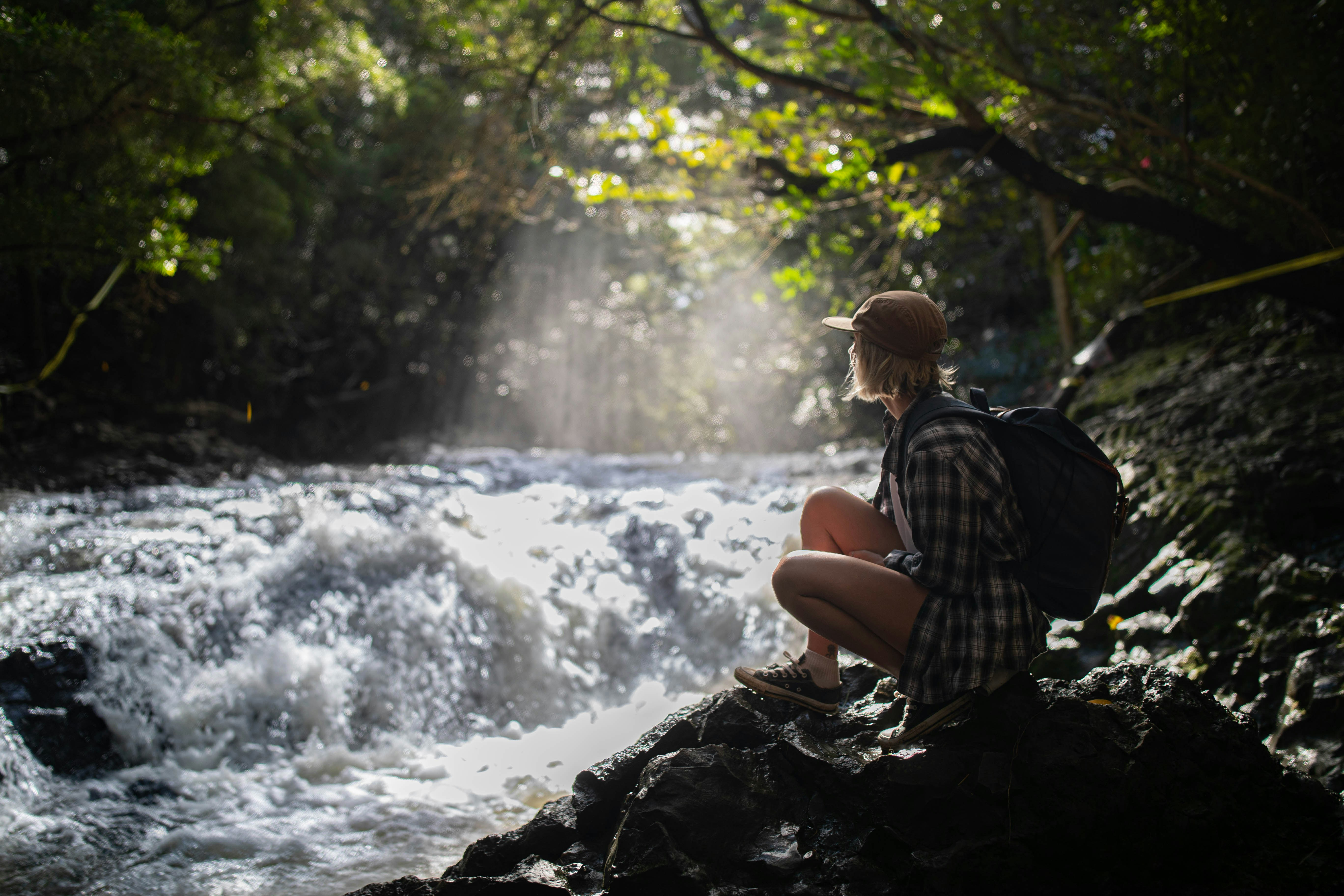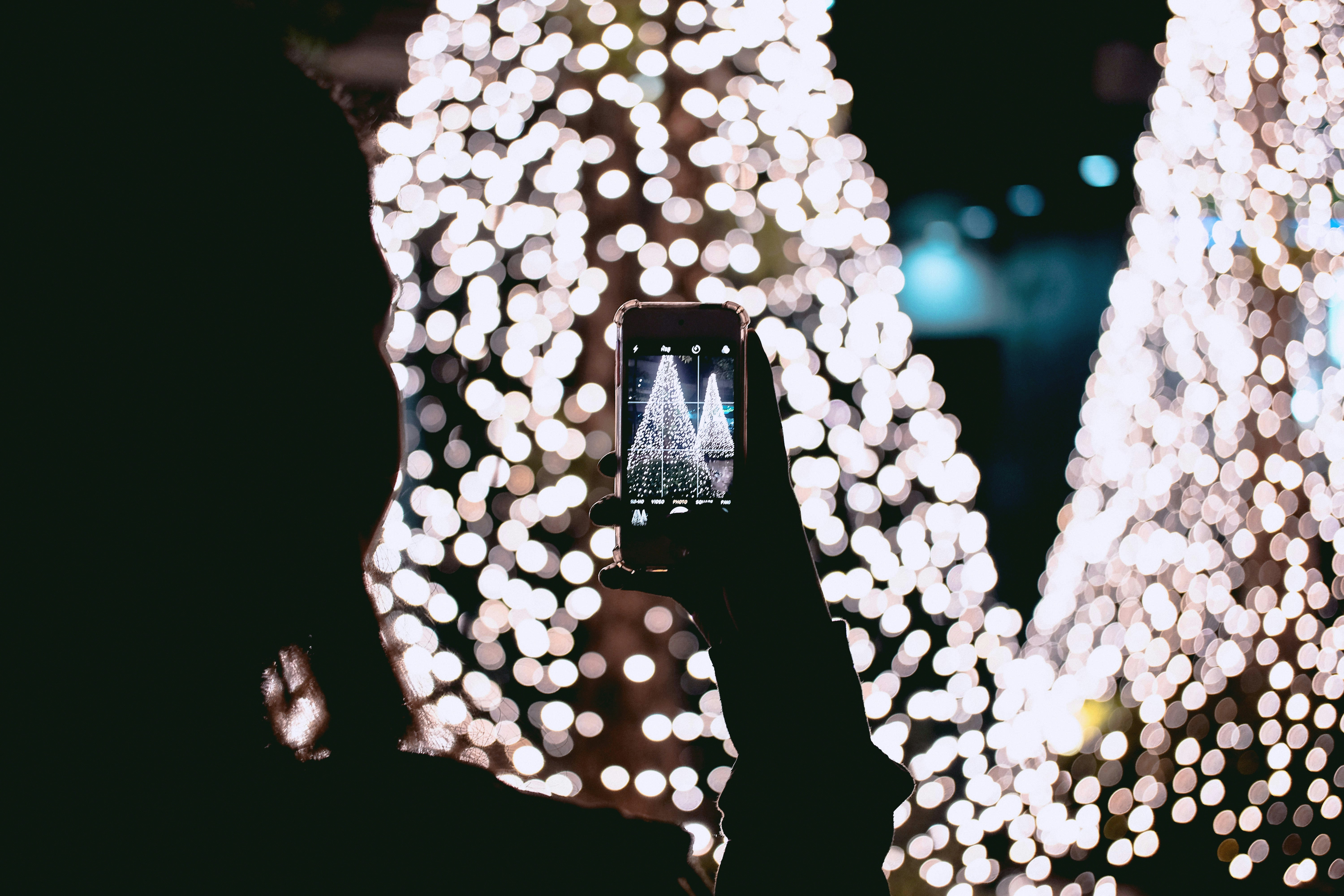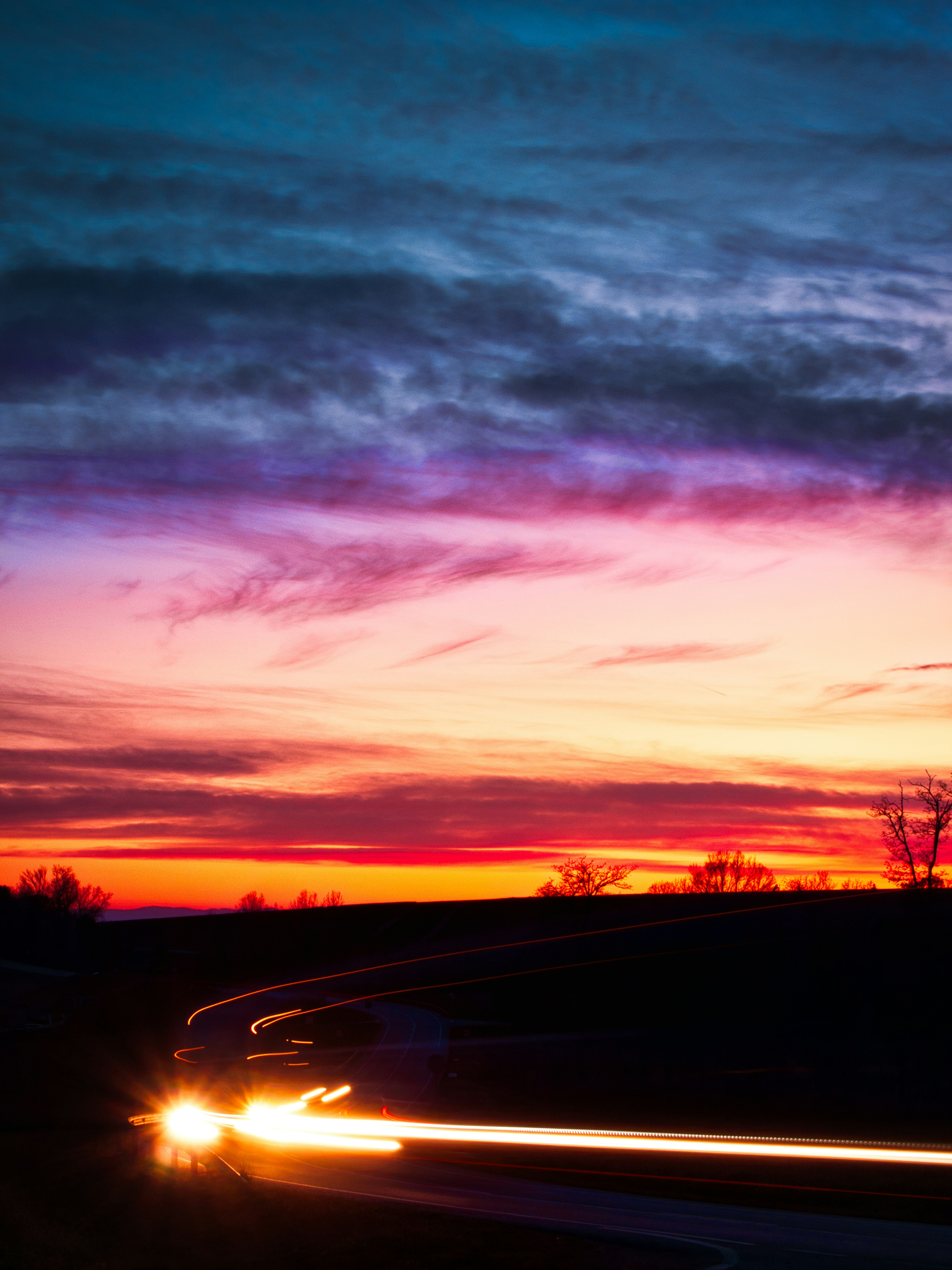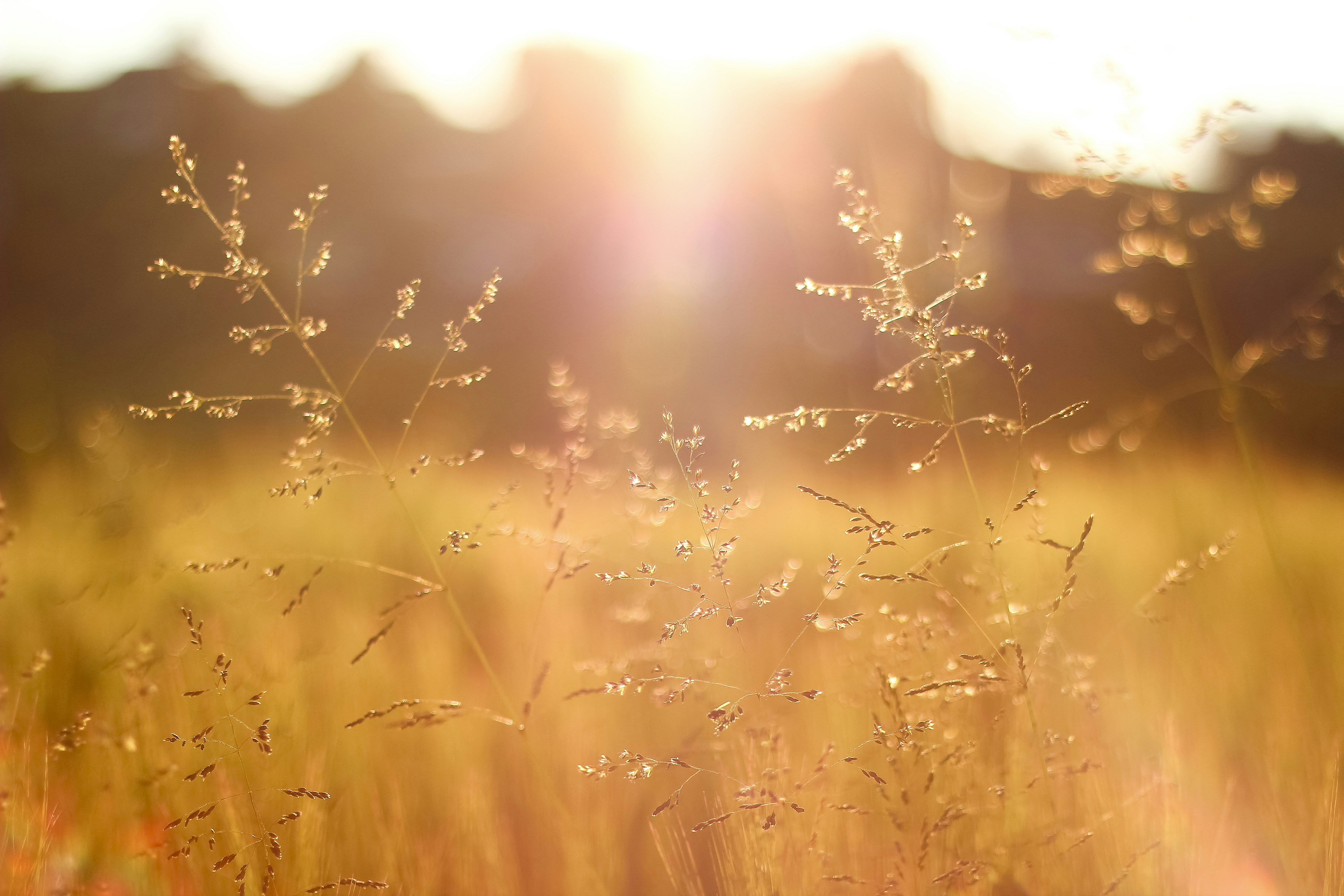## JPG Light Value Analysis with Python, PIL and MatPlotLib

Building a Histogram to analyze the light values of an image

All images used in this post are from the amazing Unsplash.com

## Introduction

We'll be making a histogram using `matplotlib` to display light distribution of pixel count in JPG images. Each pixel has an RGB value(red, green, blue) ranging 0 to 255, with the light value representing the sum of those values. `(0,0,0)` is black - zero light, and `(255,255,255)` is white - full light. Our `x` axis range will be 0 to 765.

For example - The light distribution of the this image ...is this -We can see a large distribution of dark pixels than light ones.

Why are we doing this? Because we can! While I don't have a ton of specific use cases for this, being able to use data to answer questions is important. Our initial question is "What is the light distribution of this image?"

## What we'll be doing?

All of the following steps are in Python.

1. Use `PIL` to load an image into memory.
2. Shrink the image down to a pixel size we can more easily view.
3. Use `numpy` to convert our image into an array. Flatten the 3d array into a 2d array of the RGB values.
4. Convert the pixel array into an array of the pixel light values - the sun of the rgb values.
5. Use `matplotlib` to generate the histogram.

Let's get started!

## Use PIL to load an image into memory.

PIL is an absolutely magical package for image processing. I created the `getImageFromUrl(url)` method that takes in a url, uses python's `requests` package to make the https request, and then load the image. We need to pass the response content into BytesIO to read the requests content into a format that PIL can consume and convert into an Image object.

By the end of this code, we have an image from the internet in memory as a PIL.Image object.

``````from PIL import Image
import requests

def getImageFromUrl(url):
response = requests.get(url)
return Image.open(BytesIO(response.content))

imageUrl = "https://images.unsplash.com/photo-1583364481915-dacea3e06d18?ixlib=rb-1.2.1&ixid=eyJhcHBfaWQiOjEyMDd9&auto=format&fit=crop&w=600&q=80"

image = getImageFromUrl(imageUrl)
``````

## Shrink the image down to a pixel size we can more easily view.

I created a helper method to resize the image file so that it's largest side is a pixel count we pass in. This is to keep pixel count low enough to analyze quickly and in a controlled way. By the end of this block, we have a resized image with 150 pixels as the largest side, and the aspect ratio remaining the same.

``````def resize_setLargestSide(image,maxSide):
width,height = image.size
widthRatio = width / (width + height)
heightRatio = height / (width + height)
if width > height:
newWidth = maxSide
widthPlusHeight = newWidth / widthRatio
newHeight = widthPlusHeight - newWidth
else:
newHeight = maxSide
widthPlusHeight = newHeight / heightRatio
newWidth = widthPlusHeight - newHeight
return image.resize((int(newWidth),int(newHeight)))

newImage = resize_setLargestSide(image,150)
``````

## Use `numpy` to convert our image into an array. Flatten the 3d array into a 2d array of the RGB values.

the `np.array` method converts a PIL.Image object to a 3d np array - height by width by pixels (r,g,b). numpy arrays have the property `shape`, which in the case below returns the width, height, and 3, which is the length of the pixel. I create `flattenedShape` which will be used to convert the 3d array into a 2d array by multiplying the length by width, which is then passed into `reshape()`, a method that lives on the np array.

`reshape()` only works if the number of values remains the same, so had I not multiplied width by height, `reshape()` would have failed.

``````import numpy as np

imageArray = np.array(newImage)
shape = imageArray.shape
flattenedShape = (shape * shape,shape)
reshapedImage = imageArray.reshape(flattenedShape)
``````

## Convert the pixel array into an array of the pixel light values - the sun of the rgb values.

Boy do I love list comprehensions. Below takes the 2d array and converts it to a 1 dimensional array of pixel light values, by summing the 3 values of the pixel. At this point, we have our data ready to graph!

``````lightValues = [sum(pixel) for pixel in reshapedImage]
``````

## Use `matplotlib` to generate the histogram.

And now, we graph!

``````import matplotlib.pyplot as plt

plt.hist(lightValues, bins=20, facecolor = 'blue')
plt.ylabel("Amount of Light")
plt.xlabel("Pixel Concentration")
plt.title('Light Values')
plt.axis([0,775,0,4000])
plt.show()
``````

## Full Code

``````from PIL import Image
from io import BytesIO
import requests

def getImageFromUrl(url):
response = requests.get(url)
return Image.open(BytesIO(response.content))

imageUrl = "https://images.unsplash.com/photo-1583364481915-dacea3e06d18?ixlib=rb-1.2.1&ixid=eyJhcHBfaWQiOjEyMDd9&auto=format&fit=crop&w=600&q=80"

image = getImageFromUrl(imageUrl)

def resize_setLargestSide(image,maxSide):
width,height = image.size
widthRatio = width / (width + height)
heightRatio = height / (width + height)
if width > height:
newWidth = maxSide
widthPlusHeight = newWidth / widthRatio
newHeight = widthPlusHeight - newWidth
else:
newHeight = maxSide
widthPlusHeight = newHeight / heightRatio
newWidth = widthPlusHeight - newHeight
return image.resize((int(newWidth),int(newHeight)))

newImage = resize_setLargestSide(image,150)

import numpy as np

imageArray = np.array(newImage)
shape = imageArray.shape
flattenedShape = (shape * shape,shape)
reshapedImage = imageArray.reshape(flattenedShape)

lightValues = [sum(pixel) for pixel in reshapedImage]

import matplotlib.pyplot as plt

plt.hist(lightValues, bins=20, facecolor = 'blue')
plt.ylabel("Amount of Light")
plt.xlabel("Pixel Concentration")
plt.title('Light Values')
plt.axis([0,775,0,4000])
plt.show()
``````

## Example Outputs

#### input#### output#### input#### output#### input#### output